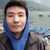## TyroCity is a community of amazing learners

We're a place where students share, stay up-to-date, learn and grow.

# Discussion on: How can a positive NPV end up with negative NPV with the same set of cash flow and time horizonNet Present Value (NPV) has practical implication and so widely used in business decision making as it includes the time value of money. This theoretical method has been found to be used 80% in business decision for new expansion and 60% for mutually exclusive projects. Its use has increased over the years for capital budgeting and financial decision making. (Sun & Queyranne, 2002) Here we will look into how positive and negative NPV are generated and what is the driving factor for this.

Let us take the following for a project.

Initial investment or Present value of cash outflow = Rs. 250,000.00

Discounted Rate of return = 10 %

Period = 5 years

Expected annual cash inflow = Rs. 70,000

In this example we are taking an example of a consistent cash flow only. Let us calculate the discounted cash inflow and net present value.

Figure 1:

A B C=A*B D=D-C
Year Cash Flow (CF) in Rs. Present Value (PV ) = 1/(1+i)^n PV of Cash inflow (in Rs) = CF * PV NPV = PV of Cash Inflow – Cash outflow (In Rs)
0 (250,000) 1 (250,000) (250,000)
1 70,000 0.9091 63,637 (186,363)
2 70,000 0.8264 57,848 (128,515)
3 70,000 0.7513 52,591 (75,924)
4 70,000 0.6830 47,810 (28,114)
5 70,000 0.6209 43,463 15,349

Here we can see that the project can be selected as it gives a positive value in the last year.

Now let us consider the same project with the same initial outflow, period, and expected cash inflow but increase the discounted rate of return to 20%.

Figure 2:

A B C=A*B D=D-C
Year Cash Flow (CF) in Rs. Present Value (PV ) = 1/(1+i)^n PV of Cash inflow (in Rs) = CF * PV NPV = PV of Cash Inflow – Cash outflow (In Rs)
0 (250,000) 1 (250,000) (250,000)
1 70,000 0.8333 58,331 (191,669)
2 70,000 0.6944 48,608 (143061)
3 70,000 0.5787 40,509 (102552)
4 70,000 0.4823 33,761 (68,791)
5 70,000 0.4019 28,133 (40,658)

In Figure 2 we can see that the NPV is still negative in the fifth year with a discounted rate of return of 20% but a positive NPV is reflected in figure 1 with a discounted rate of 10%. What this shows is that the investment in figure 2 should be rejected while the investment in figure 1 should be selected as it has positive cash flow at the end of the expected year.

From this we deduce that a higher discounted rate of return is not favourable for investment.

Reference

Sun, D., & Queyranne, M. (2002). Production and inventory model using net present value. Operations Research; Linthicum , 528-537. Retrieved from proxy.lirn.net/MuseProxyID=mp02/Mu...